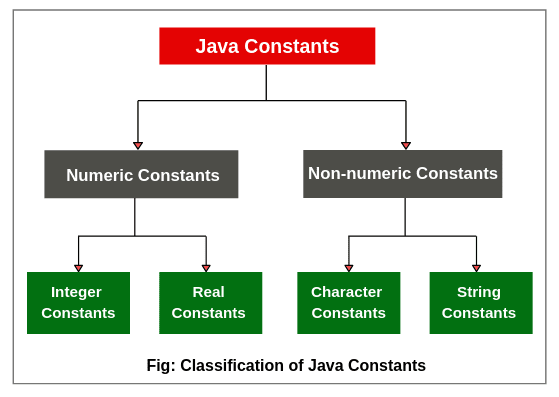# Constants in Java | Types, Example

A value which is fixed and does not change during the execution of a program is called constants in java.

In other words, Java constants are fixed (known as immutable) data values that cannot be changed.

Java supports various types of constants. They are as follows:

1. Integer Constants
2. Real Constants
3. Character Constants
4. String Constants## Integer Constants in Java

An integer constant is a sequence of digits without a decimal point. For example, 10 and -200 are integer constants. There are three types of integer constants. They are as follows:

1. Decimal integer
2. Octal integer

### Decimal Integer:

Decimal integer is a sequence of digits from 0 to 9. These constants are either positive or negative. Plus sign is optional.

For example, 24, -55, 0, and +3425 are valid examples of decimal integer constants in decimal notation.

Non-digit characters, embedded spaces, and commas are not allowed between digits. For example,  12 3450, 20.00, \$1200 are illegal numbers.

### Octal Integer:

An octal integer constant is a sequence of any combination of digits from 0 to 7. Octal integer always starts with 0. The digits 8 and 9 are not valid in octal notation.

The examples of valid integer constants in octal notation are 024, 0, 0578, and 0123.

Hexadecimal integer constants consist of digits 0 to 9 and alphabets “a” to “f” or “A” to “F”. These constants are preceded by 0x. Letter “A” to “F” represents the numbers 10 to 15.

The valid examples of hexadecimal integer constants are 0x23, 0x5B, 0x9F, 0x, etc. “x” can also be either small or capital.

We rarely use octal and hexadecimal integer numbers system in programming.

Let’s create a program to study decimal, octal, and hexadecimal notation for integers.

Program code 1:

```package constantsPrograms;
public class IntegerConst
{
public static void main(String[] args)
{
int a, b, c;
a = 20; // decimal notation.
b = 020; // octal notation.
c = 0x20F; // hexadecimal notation.

System.out.println("Decimal notation 20: " +a);
System.out.println("Octal notation 020: " +b);
}
}```
```Output:
Decimal notation 20: 20
Octal notation 020: 16

## Real (Floating-point) Constants in Java

Real constants consist of a sequence of digits with fractional parts or decimal points. These constants are also called floating-point constants. The valid examples of real constants are 2.3, 0.0034, -0.75, 56.7, etc.

These numbers are shown in the decimal point notation. It is also possible that the number may not have digits before decimal point or digits after the decimal point. For example, 205., .45, -.30, etc.

A real constants number can also be expressed in exponential or scientific notation. For example, the value 235.569 can also be written as 2.35569e2 in exponential notation. Here, e2 means multiply by 10^2.

It has the following general form:

`mantissa e exponent`

Where,

mantissa is either integer number or a real number expressed in decimal notation. The term exponent is an integer with an optional plus or minus sign.

The letter “e” is used to separate mantissa and exponent which can be written in either lowercase or uppercase. The valid examples of floating points constants are 0.54e2, 12e-4, 1.5e+5, 2.13E4, -1.5E-4, etc.

Embedded (blank) space is not permitted in any numeric constants. Exponential notation is useful to represent either very small or very large number in magnitude.

For example, 250000000 can also be written as 2.5e8 or 25E7. Similarly, -0.000000021 is equivalent to -2.1e-8.

## Single Character Constants in Java

A single character constant ( or simply character constant) is a single character enclosed within a pair of single quote. The example of single-character constants are as follows:

‘5’   ‘x’   ‘;’   ‘ ‘   etc.

The last constant is blank space. The character constant ‘5’ is not equivalent to the number 5.

## String Constants

A string constant is a sequence of characters within a pair of double-quotes. The characters can be alphabets, special characters, digits, and blank spaces. The valid examples of string constants are given below:

“Hello Java”   “1924”   “?…!”   “2+7”   “X”   etc.

## Backslash Character Constants

Java provides some special backslash character constants that are used in output methods. For example, the symbol ‘n’ which stands for newline character.

There are various types of backslash character constants that are supported by java. The list is given in the below table.

Table: Backslash Character Constants

ConstantsMeaning
‘ b ‘back space
‘ f ‘form feed
‘ n ‘new line
‘ r ‘carriage return
‘ t ‘horizontal tab
‘ ‘ ‘single quote
‘ ” ‘double quote
‘  ‘backslash

Hope that this tutorial has covered almost all important points related to constants in java with example programs. I hope that you will have understood this tutorial and enjoyed it.